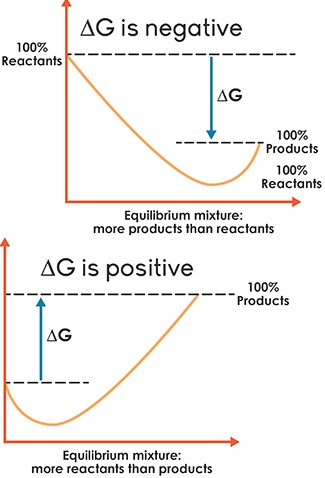## Law of Mass Action

In 1864 two Norwegian chemists namely Maximilian Guldberg and Peter Waage formulated the law of mass action, based on the experimental studies of many reversible reactions.

The law states that, “At any instant, the rate of a chemical reaction at a given temperature is directly proportional to the product of the active masses of the reactants at that instant”.

Rate α [Reactant]x

where, x is the stoichiometric coefficient of the reactant and the square bracket represents the active mass (concentration) of the reactants.

Active mass = ($$\frac{n}{V}$$) mol dm-3(or) mol L-1

where n is the number of moles and V is the volume of the container (dm3 or L)Equilibrium constants (Kp and Kc):

Let us consider a reversible reaction,

xA + yB ⇄ lC + mD

where, A and B are the reactants, C and D are the products and x, y, l and m are the stoichiometric coeffients of A, B, C and D, respectively.

Applying the law of mass action, the rate of the forward reaction,

rfα[A]x[B]y (or) rf = kf[A]x[B]y

Similarly, the rate of the backward reaction,

rbα[C]l[D]m
(or)
rb=Kb[C]l[D]m

where kf and kb are proportionality constants

At equilibrium,

Rate of forward reaction (rf)
= Rate of backward reaction (rb)
kf[A]x[B]y = kb[C]l[D]m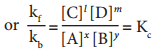where, Kc is the equilibrium constant in terms of concentration (active mass).

At a given temperature, the ratio of the product of active masses of reaction products raised to the respective stoichiometric coefficients in the balanced chemical equation to that of the reactants is a constant, known as equilibrium constant. Later when we study chemical kinetics we will learn that this is only approximately true.

If the reactants and products of the above reaction are in gas phase, then the equilibrium constant can be written in terms of partial pressures as indicated below,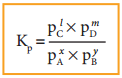Where, pA, pB, pC, and pD are the partial pressures of the gas A, B, C and D, respectively.Relation Between Kp and Kc

Let us consider the general reaction in which all reactants and products are ideal gases.

xA + yB ⇄ lC + mD

The equilibrium constant, Kc is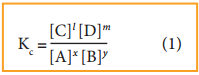and Kp is,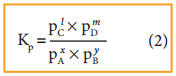The ideal gas equation is

PV = nRT
or
P = $$\frac{n}{V}$$RT

Since

Active mass = molar concentration = n/V
P = active mass × (RT)

Based on the above expression the partial pressure of the reactants and products can be expressed as,

PxA = [A]x(RT)x
PyB = [B]y(RT)y
Plc = [C]l(RT)l
PmD = [D]m(RT)m

On substitution in Eqn.2,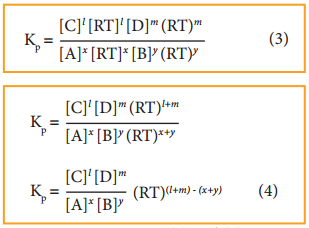By comparing equation (1) and (4), we get

Kp = kc(RT)∆ng ………… (5)

where, Δng is the difference between the sum of number of moles of products and the sum of number of moles of reactants in the gas phase.

The following relations become immediately obvious.

When Δng = 0
Kp = Kc(RT)°
Kp = KcExamples:

1. H2(g) + I2(g) ⇄ 2HI(g)
2. N2(g) + O2(g) ⇄ 2NO(g)

When Δng = +ve
Kp = Kc(RT)+ve
Kp > kc(RT)

Examples:

1. 2NH3(g) ⇄ N2(g) + 3H2(g)
2. PCI5(g) ⇄ PCl3(g) + Cl2(g)

When Δng = – ve
KP = KC(RT)-ve
KP < KC

Examples:

1. 2H2(g) + O2(g) ⇄ 2H2O(g)
2. 2SO2(g) + O2(g) ⇄ 2SO3(g)

Relation Between Equilibrium Constants for Some Reversible Reactions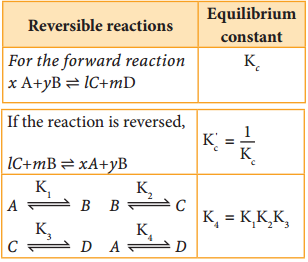Equilibrium Constants for Heterogeneous Equilibrium

Consider the following heterogeneous equilibrium.

CaCO3(s) ⇄ CaO(s) + CO2(g)

The equilibrium constant for the above reaction can be written as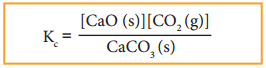A pure solid always has the same concentration at a given temperature, as it does not expand to fill its container. i.e. it has same number of moles L-1 of its volume. Therefore, the concentration of a pure solid is a constant. The above expression can be modified as follows.

KC = [CO2]
or
KP = PCO2

The equilibrium constant for the above reaction depends only the concentration of carbon dioxide and not the calcium carbonate or calcium oxide. Similarly, the active mass (concentration) of the pure liquid does not change at a given temperature. Consequently, the concentration terms of pure liquids can also be excluded from the expression of the equilibrium constant.

For example,

CO2(g) + H2O(l) ⇄ H+(aq) + HCO3(aq)
Since, H2O((l)) is a pure liquid the Kc can be expressed as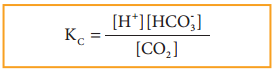Example

Write the KP and Kc for the following reactions

1. 2SO2(g) + O2(g) ⇄ 2SO3(g)
2. 2CO(g) ⇄ CO2(g) + C(s)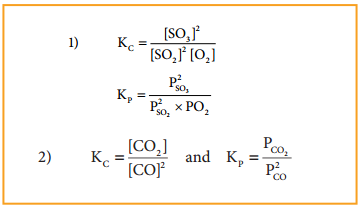Find free online Chemistry Topics covering a broad range of concepts from research institutes around the world.

## Homogeneous and Hetrogeneous Equilibrium

Find free online Chemistry Topics covering a broad range of concepts from research institutes around the world.

## Homogeneous and Hetrogeneous Equilibrium

In a homogeneous equilibrium, all the reactants and products are in the same phase.
For example

H2(g) + I2(g) ⇄ 2HI(g)

In the above equilibrium, H2, I2 and HI are in the gaseous state.

Similarly, for the following reaction, all the reactants and products are in homogeneous solution phase.

CH3COOCH3(aq) + H2O(aq)
↑↓
Heterogeneous Equilibrium

If the reactants and products of a reaction in equilibrium, are in different phases, then it is called as heterogeneous equilibrium.

Example:

H2O(l) ⇄ H2O(g)
CaCO3(s) ⇄ CaO(s) + CO2(g)

A homogeneous equilibrium is one in which all species are present in the same phase. Common examples include gas-phase or solution reactions. A heterogeneous equilibrium is one in which species exist in more than one phase. Common examples include reactions involving solids and gases, or solids and liquids.A homogenous equilibrium reaction is a reaction where all of the products and reactants are in the same phase. The reactants are on the left side of the arrows, and the products are on the right side of the arrows. A heterogeneous equilibrium reaction is when there are different phases in the reaction.

Homogeneous reactions are chemical reactions in which the reactants and products are in the same phase, while heterogeneous reactions have reactants in two or more phases. A reaction between a gas and a liquid, a gas and a solid or a liquid and a solid is heterogeneous.

In an equilibrium, the reactants and the products are present in two or more that two phases, it is called a heterogeneous equilibrium.

A heterogeneous equilibrium is one in which species exist in more than one phase. Common examples include reactions involving solids and gases, or solids and liquids.By definition, a pure substance or a homogeneous mixture consists of a single phase. A heterogeneous mixture consists of two or more phases. When oil and water are combined, they do not mix evenly, but instead form two separate layers. Each of the layers is called a phase.

Homogeneous solutions are solutions with uniform composition and properties throughout the solution. For example a cup of coffee, perfume, cough syrup, a solution of salt or sugar in water, etc. Heterogeneous solutions are solutions with non-uniform composition and properties throughout the solution.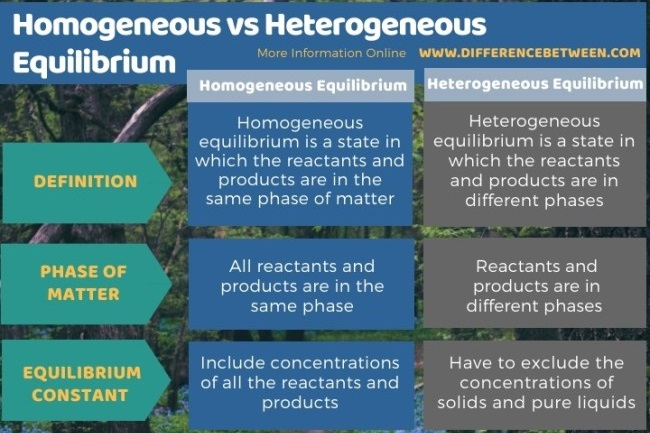## Chemical Equilibrium

Find free online Chemistry Topics covering a broad range of concepts from research institutes around the world.

## Chemical Equilibrium

Similar to physical processes chemical reactions gradually attain a state of equilibrium after sometime. Let us consider a general reversible reaction.

A + B ⇄ C + D

Initially only A and B are present Soon, a few molecules of the products C and D are formed by the forward reaction. As the concentration of the products increases, more products collide and react in the backward direction. This leads to an increase in the rate of backward reaction. As the rate of reverse reaction increases, the rate of the forward reaction decreases. Eventually, the rate of both reactions becomes equal.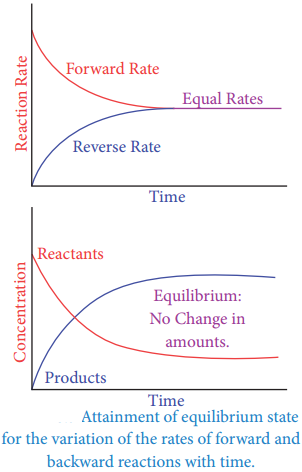Let us consider, a situation in multi storey building, people are moving from first floor to second flor and vice versa. Assume that a certain number people moves up from first floor to second floor in a specific time, and the same number of people moves down from second floor to the first floor in the same time.Now, the rate of movement of people from first to second floor equals the rate of movement of people from second to first floor, and hence the number of people in each floor will remain the same. This the population of people on the two floors is in a dynamic equilibrium Let us extend this analogy to understand dynamic nature of equilibrium.

Chemical reactions which are reversible do not cease, when equilibrium is attained. At equilibrium the forward and the backward reactions are proceeding at the same rate and no macroscopic change is observed. So chemical equilibrium is in a state of dynamic equilibrium.

For example,

H2(g) + I2(g) ⇄ 2HI (g)

## Physical and Chemical Equilibrium

Find free online Chemistry Topics covering a broad range of concepts from research institutes around the world.

## Physical and Chemical Equilibrium

There are different types of equilibrium. For example, if two persons with same weight sit on opposite sides of a see-saw at equal distance from the fulcrum, then the see-saw will be stationary and straight and it is said to be in equilibrium.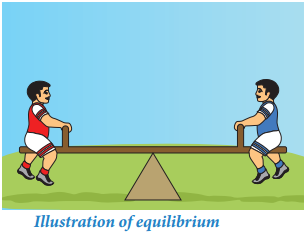Another example of a state of equilibrium is the game of “tug-of-war.” In this game a rope is pulled taut between two teams. There may be a situation when both the teams are pulling the rope with equal force and the rope is not moving in either direction. This state is said to be in equilibrium.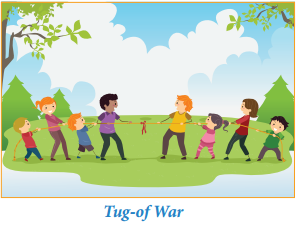In reversible processes, the rate of two opposing reactions equals at a particular stage. At this stage the concentration of reactants and products do not change with time. This condition is not static and is dynamic, because both the forward and reverse reactions are still occurring with the same rate.Physical Equilibrium

A system in which the amount of matter constituting different phases does not change with time is said to be in physical equilibrium. This involves no perceptible physical change in the system. To understand the physical equilibrium let us analyse the following phase changes.

Solid-liquid Equilibrium

Let us consider the melting of ice in a closed container at 273 K. This system will reach a state of physical equilibrium in which the amount of water in the solid phase and liquid phase does not change with time. In the process the total number of water molecules leaving from and returning to the solid phase at any instant are equal.

If some ice-cubes and water are placed in a thermos flask (at 273K and 1 atm pressure), then there will be no change in the mass of ice and water.

At equilibrium,

Rate of melting of ice = Rate of freezing

H2O ⇄ H2O(l)

The above equilibrium exists only at a particular temperature and pressure. The temperature at which the solid and liquid phases of a substance are at equilibrium is called the melting point or freezing point of that substance.

Liquid – Vapour equilibrium

Similarly, there exists an equilibrium between the liquid phase and the vapour phase of a substance. For example, liquid water is in equilibrium with its vapour at 373 K and 1 atm pressure in a closed vessel.

H2O(l) ⇄ H2O(g)

Here

Rate of evaporation = Rate of condensation

The temperature at which the liquid and vapour phases of a substance are at equilibrium is called the boiling point and condensation point of that substance.Solid – Vapour Equilibrium

Consider a closed system in which the solid sublimes to vapour. In this process also, equilibrium can be established between these two phases. When solid iodine is placed in a closed transparent vessel, after sometime,the vessel gets filled up with violet vapour due to sublimation of iodine.

Initially, the intensity of the violet colour increases, after sometime it decreases and finally it becomes constant, as the following equilibrium is attained.

I2(s) ⇄ I2(g)

More Examples

Camphor (s) ⇄ Camphor (g)
NH4Cl(s) ⇄ NH4Cl(g)

Equilibrium Involving Dissolution of Solids or Gases in liquids

Solid in liquids

When you add sugar to water at a particular temperature, it dissolves to form sugar solution. If you continue to add much sugar, you will reach a stage at which the added sugar remains as solid and the resulting solution is called a saturated solution. Here, as in the previous cases a dynamic equilibrium is established between the solute molecules in the solid phase and in the solution phase.

Sugar (Solid) ⇄ Sugar (Solution)

In this process

Rate of dissolution of solute = Rate of crystallisation of soluteGas in liquids

When a gas dissolves in a liquid under a given pressure, there will be an equilibrium between gas molecules in the gaseous state and those dissolved in the liquid.

Example:

In carbonated beverages the following equilibrium exists.
CO2(g) ⇄ CO2(Solution)

Henry’s law is used to explain such gas-solution equilibrium processes.

## Introduction of Equilibrium

Find free online Chemistry Topics covering a broad range of concepts from research institutes around the world.

## Introduction of Equilibrium

In our daily life, we observe several chemical and physical changes. For example, a banana gets ripened after few days, silver gets tarnished in few months and iron gets rusted slowly. These processes proceed in one direction.

Now let us consider the transport of oxygen by hemoglobin in our body as an illustration for a reversible change. The hemoglobin combines with oxygen in lungs to form oxyhemoglobin. The oxyhemoglobin has a tendency to form hemoglobin by releasing oxygen. In fact, in our lungs all the three species coexist.

Few chemical reactions proceed in only one direction whereas many reactions proceed in both the directions and these reactions are called reversible reactions. In chemical reactions, the concentration of the reactants decreases and that of the products increases with time. In reversible reactions, initially the reaction proceeds towards the formation of the product.

Upon formation of the product, the reverse reaction begins to take place. At a particular stage, the rate of the reverse reaction is equal to that of the forward reaction indicating a state of equilibrium. It is desirable to know the three crucial aspects of chemical reactions namely the feasibility, the rate of the reaction and the extent of reaction.We know that the feasibility of a reaction is given by thermodynamics. Chemical kinetics will tell about the rate of the reaction. The equilibrium constant tells about the extent of a reaction which we will discuss in this chapter. We will also discuss the types of equilibrium, the significance of equilibrium constant and its relationship to thermodynamic quantities and the response of chemical equilibrium to change in the reaction conditions.

At equilibrium, with both the forward and reverse reactions taking place at the same rate, the concentration of every species no longer changes. Every reaction has a point in which equilibrium is established. But for other reactions, equilibrium occurs when only part of the reactants are converted into products.

Equilibrium is the state in which market supply and demand balance each other, and as a result prices become stable. Generally, an over-supply of goods or services causes prices to go down, which results in higher demand-while an under-supply or shortage causes prices to go up resulting in less demand.

A state of rest or balance due to the equal action of opposing forces. equal balance between any powers, influences, etc.; equality of effect. mental or emotional balance; equanimity: The pressures of the situation caused her to lose her equilibrium.

Equilibrium always happens at the same point in the reaction no matter where you start. They reach equilibrium at the same point whether you start with all A, all B/C, or half A and half B/C. It doesn’t matter. There is one special point where the forward and reverse reactions cancel each other out.

Equilibrium is important to create both a balanced market and an efficient market. If a market is at its equilibrium price and quantity, then it has no reason to move away from that point, because it’s balancing the quantity supplied and the quantity demanded.

Another way of defining equilibrium is to say that a system is in equilibrium when the forward and reverse reactions occur at equal rates. Equilibrium does not necessarily mean that reactants and products are present in equal amounts.An example of equilibrium is when you are calm and steady. An example of equilibrium is when hot air and cold air are entering the room at the same time so that the overall temperature of the room does not change at all.

A fish is an ideal example of equilibrium. A fish swimming at a certain depth under the sea without floating upward or sinking unexpectedly is said to be in equilibrium. This is achieved by the swim bladder present in it. The bladder is present in the belly of a fish.

Explanation:

A mixture is a substance in which two or more components are mixed together. For example, sugar in water, mud in water etc are all mixture. Whereas an equilibrium mixture is a mixture in which components of both reactants and products are present in ratio with each other.

Equilibrium is important to create both a balanced market and an efficient market. If a market is at its equilibrium price and quantity, then it has no reason to move away from that point, because it’s balancing the quantity supplied and the quantity demanded.